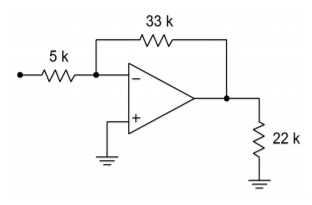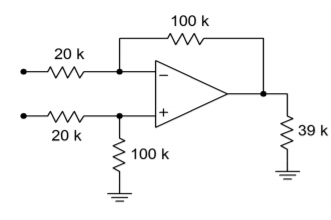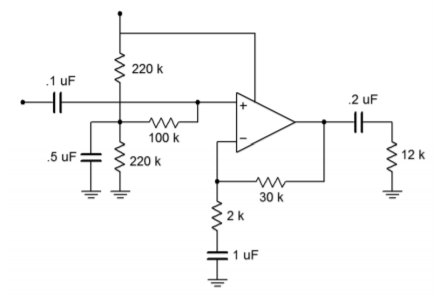# 7.10.6: Problems

$$\newcommand{\vecs}{\overset { \rightharpoonup} {\mathbf{#1}} }$$ $$\newcommand{\vecd}{\overset{-\!-\!\rightharpoonup}{\vphantom{a}\smash {#1}}}$$$$\newcommand{\id}{\mathrm{id}}$$ $$\newcommand{\Span}{\mathrm{span}}$$ $$\newcommand{\kernel}{\mathrm{null}\,}$$ $$\newcommand{\range}{\mathrm{range}\,}$$ $$\newcommand{\RealPart}{\mathrm{Re}}$$ $$\newcommand{\ImaginaryPart}{\mathrm{Im}}$$ $$\newcommand{\Argument}{\mathrm{Arg}}$$ $$\newcommand{\norm}{\| #1 \|}$$ $$\newcommand{\inner}{\langle #1, #2 \rangle}$$ $$\newcommand{\Span}{\mathrm{span}}$$ $$\newcommand{\id}{\mathrm{id}}$$ $$\newcommand{\Span}{\mathrm{span}}$$ $$\newcommand{\kernel}{\mathrm{null}\,}$$ $$\newcommand{\range}{\mathrm{range}\,}$$ $$\newcommand{\RealPart}{\mathrm{Re}}$$ $$\newcommand{\ImaginaryPart}{\mathrm{Im}}$$ $$\newcommand{\Argument}{\mathrm{Arg}}$$ $$\newcommand{\norm}{\| #1 \|}$$ $$\newcommand{\inner}{\langle #1, #2 \rangle}$$ $$\newcommand{\Span}{\mathrm{span}}$$$$\newcommand{\AA}{\unicode[.8,0]{x212B}}$$

## 4.6.1: Review Questions

1. What forms of feedback are used for the inverting and noninverting voltage amplifiers?

2. What forms of feedback are used for the current-to-voltage and voltage-to-current transducers?

3. What form of feedback is used for the inverting current amplifier?

4. What are the op amp analysis idealizations?

5. What is virtual ground?

6. What is a summing amplifier?

7. How can output current by increased?

8. What circuit changes are needed in order to bias an op amp with a unipolar supply?

9. What operational parameters change when a circuit is set up for single supply biasing?

10. How might a circuit's gain be controlled externally?

11. What is meant by the term “floating load”?

## 4.6.2: Problems

### Analysis Problems

1. What is the voltage gain in Figure $$\PageIndex{1}$$? What is the input impedance?Figure $$\PageIndex{1}$$

2. What is the voltage gain for the first stage of Figure $$\PageIndex{2}$$? What is the input impedance?Figure $$\PageIndex{2}$$

3. What is the voltage gain for the second stage of Figure $$\PageIndex{2}$$? What is the input impedance?

4. What is the system voltage gain in Figure $$\PageIndex{2}$$? What is the input impedance?

5. If the input to Figure $$\PageIndex{2}$$ is -52 dBV, what is $$V_{out}^{'}$$ ?

6. What is the voltage gain in Figure $$\PageIndex{3}$$? What is the input impedance?

7. If the input voltage to the circuit of Figure $$\PageIndex{3}$$ is 100 mV, what is $$V_{out}$$?Figure $$\PageIndex{3}$$

8. What is the system input impedance in Figure $$\PageIndex{4}$$? What is the system gain?Figure $$\PageIndex{4}$$

9. Redesign Figure $$\PageIndex{4}$$ for an input impedance of 20 k$$\Omega$$.

10. Given an input current of 2 $$\mu$$A, what is the output voltage in Figure $$\PageIndex{5}$$?Figure $$\PageIndex{5}$$

11. What is the meter deflection in Figure $$\PageIndex{6}$$ if the input voltage is 1 V?Figure $$\PageIndex{6}$$

12. What input voltage will produce full-scale deflection in figure $$\PageIndex{6}$$?

13. Determine a new value for the 10 k$$\Omega$$ resistor in Figure $$\PageIndex{6}$$ such that a 0.1 V input will produce full-scale deflection.

14. What is the current gain in Figure $$\PageIndex{7}$$?Figure $$\PageIndex{7}$$

15. What is the maximum input current in Figure $$\PageIndex{7}$$, assuming the circuit is running off of $$\pm$$15 V supplies, and the op amp has a maximum output current of 25 mA?

16. If the differential input signal is 300 mV in Figure $$\PageIndex{8}$$, what is $$V_{out}$$?Figure $$\PageIndex{8}$$

17. Determine new values for the voltage divider resistors in Figure $$\PageIndex{8}$$, such that the resulting input impedance is balanced.

### Design Problems

18. Design a noninverting amplifier with a voltage gain of 32 dB and an input impedance of 200 k$$\Omega$$.

19. Design a voltage follower with a gain of 0 dB.

20. Design an inverting amplifier with a voltage gain of 14 dB and an input impedance of 15 k$$\Omega$$.

21. Design a current-to-voltage transducer such that a 20 $$\mu$$A input current will produce a -1 V output.

22. Design a voltage-to-current transducer such that a 100 mV input will produce a 1 mA output.

23. Design a current amplifier with a gain of -20.

24. Design a differential amplifier with a gain of 18 dB and a balanced input impedance of 25 k$$\Omega$$ per input.

25. Design a voltage to current transducer with a transconductance of 1 mS. If $$V_{in}$$ is 200 mV, what is $$I_{out}$$?

26. Design a current to voltage transducer with a transresistance of 10 k$$\Omega$$. If the input current is 500 $$\mu$$A, what is $$V_{out}$$?

27. Redesign the circuit of Figure $$\PageIndex{1}$$ for single supply operation (don't bother calculating capacitor values).

28. Redesign the circuit of Figure $$\PageIndex{3}$$ for single supply operation (don't bother calculating capacitor values).

29. Design a summing amplifier such that channel 1 has a gain of 10, channel 2 has a gain of 15, and channel 3 has a gain of 5. The minimum channel input impedance should be 1 k$$\Omega$$.

30. Determine capacitor values for Problem 27 if the lower break frequency $$f_1$$, is set to 20 Hz.

31. Determine capacitor values for Problem 28 if the lower break frequency f1, is set to 10 Hz.

### Challenge Problems

32. Design a three channel summing amplifier such that: channel 1 $$Z_{in} \geq 10 k\Omega$$, $$A_v$$ = 6 dB; channel 2 $$Z_{in} \geq 22 k\Omega$$, $$A_v$$ = 10 dB; and channel 3 $$Z_{in} \geq 5 k\Omega$$, $$A_v$$ = 16 dB

33. Assuming 10% resistor values, determine the production gain range for Figure $$\PageIndex{1}$$.

34. Assuming 5% resistor values, determine the highest gain produced in Figure $$\PageIndex{2}$$.

35. Design an inverting amplification circuit with a gain of at least 40 dB, and an input impedance of at least 100 k$$\Omega$$. No resistor used may be greater than 500 k$$\Omega$$. Multiple stages are allowed.

36. Redesign the circuit of Figure $$\PageIndex{3}$$ as a voltmeter with 500 mV, 2 V, 5 V, 20 V, and 50 V ranges.

37. Assuming 1% precision resistors and a meter accuracy of 5%, what range of input values may produce a full-scale reading of 2 V, for the circuit of Problem 36?

38. Design an amplifier with a gain range from -10 dB to +20 dB, with an input impedance of at least 10 k$$\Omega$$.

39. Design an amplifier with a gain range from 0 to 20. The input impedance should be at least 5 k$$\Omega$$.

40. What is the input impedance in Figure $$\PageIndex{9}$$? What is $$A_v$$?Figure $$\PageIndex{9}$$

41. How much power supply ripple attenuation does the input biasing network of Figure $$\PageIndex{9}$$ produce (assume $$f_{ripple}$$ = 120 Hz)?

42. Assume that the circuit of Figure $$\PageIndex{10}$$ utilizes a standard 20 mA output op amp. If the output devices are rated for a maximum collector current of 5 amps and a Beta of 50, what is the maximum load current obtainable?Figure $$\PageIndex{10}$$

43. What are the voltage gain and input impedance in Figure $$\PageIndex{10}$$?

44. Given a summer based on Figure 4.2.22, sketch the output waveform if $$R_1 = R_2 =$$ 10 k$$\Omega$$, $$R_3 = R_f =$$ 30 k$$\Omega$$, $$R_i$$ = 15 k$$\Omega$$, $$V_1$$ = 0.3 V DC, $$V_2 = 0.1 \sin 2\pi 50 t$$ and $$V_3 = -0.2 \sin 2\pi 200 t$$.

45. Prove Equation 4.2.15 for the case when all resistors are of equal value.

### Computer Simulation Problems

46. Simulate the operation of the circuit in Figure 4.2.9. Verify the output voltage and the virtual ground at the inverting input.

47. Use a simulator to verify the maximum and minimum gains of the circuit in Figure 4.2.11.

48. Use a simulator to verify the load current and the voltage of the circuit in Figure 4.2.17.

49. Verify the output potential of the circuit in Figure 4.2.20.

50. Simulate the output voltage of the circuit of Figure $$\PageIndex{4}$$ for the following inputs:

A) $$V_{in} = 0.1$$ VDC,

B) $$V_{in}(t) = 1 \sin 2\pi 10 t$$,

C) $$V_{in} = 5$$ VDC.

Also, note the potential at the output of the first stage. How might your op amp model affect the results?

51. Simulate the circuit in Figure 4.2.27. Determine the output potential for the following inputs:

A) $$V_{in+}(t) = 0.1 \sin 2\pi 10t$$, $$V_{in-}(t) = 0.1 \sin 2\pi 10t$$,

B) $$V_{in+}(t) = 0.1 \sin 2\pi 10t$$, $$V_{in-}(t) = -0.1 \sin 2\pi 10t$$.

52. Simulate the circuit of Figure $$\PageIndex{10}$$, and determine the output of the circuit and op amp for inputs of 0.1 V DC and 1 V DC.

Use the space below creatively. Draw a cartoon. Compose a song. Write a limerick. Whatever works for you. If you're stuck, try starting with this: ♫

This page titled 7.10.6: Problems is shared under a CC BY-NC-SA license and was authored, remixed, and/or curated by James M. Fiore.